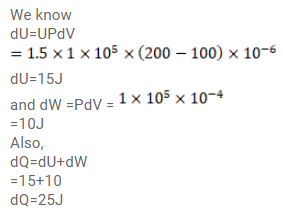# The internal energy of a gas is given byQuestion:

The internal energy of a gas is given by $\mathrm{U}=1.5 \mathrm{pV}$. It expands from $100 \mathrm{~cm}^{3}$ to $200 \mathrm{~cm}^{3}$ against a constant pressure of $1.0 \times 10^{6} \mathrm{~Pa}$ Calculate the heat absorbed by the gas in the process.

Solution: